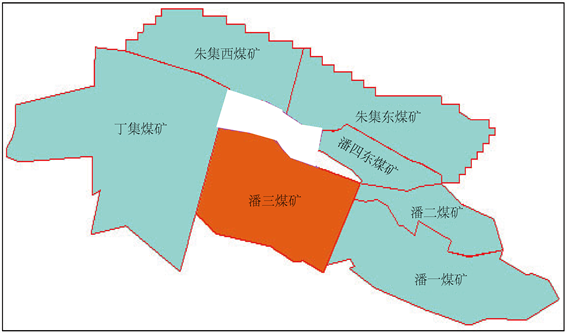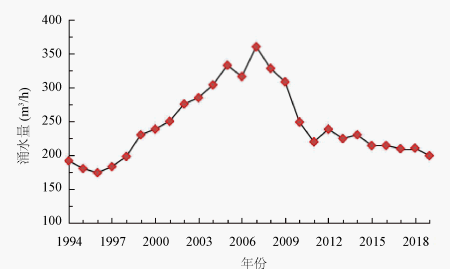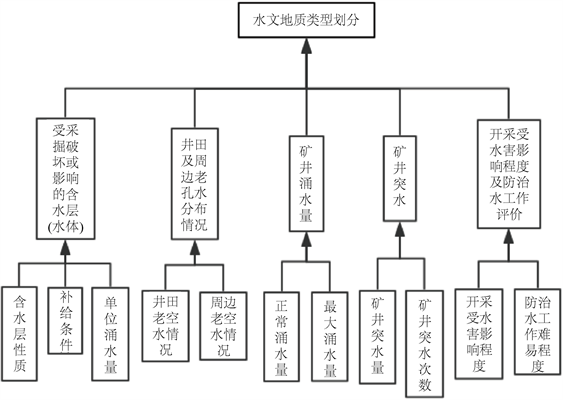﻿ 矿井水文地质类型的定性与定量划分

# 矿井水文地质类型的定性与定量划分Qualitative and Quantitative Division of Mine Hydrogeological Types

《煤矿防治水细则》中明确指出煤矿水文地质类型要每三年划分一次。针对潘三矿水文地质类型划分问题，以收集整理的潘三矿概况及近三年采掘揭露的基础水文地质资料为根本，在之前该矿编制的地质报告等报告的基础上，应用构造、水文地球化学与层次分析等相关理论，结合勘探与生产实际，按照细则中各项划分条款，详细地分析了各划分指标情况及影响程度，采用传统方法与层次分析法，从定性与定量两个角度对该矿水文地质类型进行划分。结果表明：两种方法都将水文地质类型划分为“中等”，划分结果一致，互为补证。可为潘三矿水文地质类型划分提供一种新方式，也为其矿井水害的防治工作提供了参考性意见。

Abstract: The “Detailed rules for the prevention and control of water in coal mines” clearly points out that the hydrogeological types of coal mines should be divided every three years. In view of the division of hydrogeological types of Pansan coal mine, based on the general situation and the basic hydrogeological data revealed and collected in recent three years of Pansan coal mine, on the basis of the previous geological reports and other reports prepared by the mine, applying the theories of structure, hydrogeochemistry and analytic hierarchy process, combined with the practice of exploration and production, and in accordance with the detailed rules of division, this paper analyzes in detail the situation and influence degree of each division index, and uses the traditional method and analytic hierarchy process to divide the hydrogeological types of the mine from the qualitative and quantitative angles. The results show that the two methods divide the hydrogeo-logical types into “medium”, and the division results are consistent and complementary to each other. It can provide a new way for the division of hydrogeological types of Pansan coal mine, and also provides a reference for the prevention and control of mine water disaster.

1. 引言

2. 矿井简介Figure 1. Relation diagram of adjacent mines

3. 层次分析法理论分析

1、建立需要的层次分析构型

2、构筑层次分析判断矩阵

$A={\left({a}_{ij}\right)}_{n×n}$ (1)

${a}_{ij}<\text{1}$，表示AiAj重要；

${a}_{ij}>\text{1}$，表示AiAj重要；

${a}_{ij}=\text{1}$，表示AiAj同样重要。

3、得出特征向量及指标权重值且做出一致性验证

a. 判断矩阵A各行各元素积的计算：

${m}_{i}=\underset{i=1}{\overset{n}{\prod }}{a}_{ij}\left(i=1,2,\cdots ,n\right)$ (2)

b. 计算指标权重：

${w}_{i}={\stackrel{¯}{w}}_{i}/\underset{j=1}{\overset{n}{\sum }}{\stackrel{¯}{w}}_{j}$，其中 ${\stackrel{¯}{w}}_{i}=\sqrt[n]{{m}_{i}}$ (3)

c. 对矩阵A和指标权重集作乘求出AW矩阵；

d. 最大特征值λmax (近似计算)：

${\lambda }_{\text{max}}=\underset{i=1}{\overset{n}{\sum }}{\left(AW\right)}_{i}/n{w}_{i}$ (4)

a. 求出一致性指标：

$CI=\frac{{\lambda }_{\mathrm{max}}-n}{n-1}$ (5)

b. 求出相对一致性指标：

$CR=CI/RI$ (6)

4、求出组合权重

${\stackrel{¯}{w}}_{i}={\left({\stackrel{¯}{w}}_{1},{\stackrel{¯}{w}}_{2},\cdots ,{\stackrel{¯}{w}}_{k}\right)}^{\text{T}}$ (7)

${\stackrel{¯}{w}}_{i}={\left({w}_{\text{1}i},{w}_{2i},\cdots ,{w}_{ni}\right)}^{\text{T}}$ (8)

${w}_{\text{1}}=\underset{j=1}{\overset{k}{\sum }}{w}_{j}{w}_{1j}$${w}_{\text{2}}=\underset{j=1}{\overset{k}{\sum }}{w}_{j}{w}_{\text{2}j}$ ${w}_{n}=\underset{j=1}{\overset{k}{\sum }}{w}_{j}{w}_{nj}$ (9)

5、对比综合权重值

4. 传统定性划分

4.1. 井田主要含水层概况

4.1.1. 新生界松散含水层

4.1.2. 二叠系砂岩裂隙含水层

4.1.3. 石炭系太原组灰岩岩溶裂隙含水层

4.1.4. 奥陶系灰岩岩溶裂隙含水层

4.2. 井田主要隔水层概况

4.2.1. 新生界隔水层

4.2.2. 风化带弱隔水层

4.2.3. 1煤底板隔水层

1煤底板与太原组1灰顶部的距离约为8.9~21.2 m，平均厚度16.66 m，其岩性大部分是砂泥岩与泥岩组成的互层，另含有少量的细砂岩薄层，微小裂隙不常见，因此具备一定的隔水性能。

4.3. 井田及周边地区老窑水分布状况Table 1. Workface table affected by goaf water according to mining time sequence from 2019 to 2022

4.4. 矿井涌(突)水分析

4.4.1. 矿井涌水量Figure 2. Variation curve of average water inflow in mine from 1993 to 2019

4.4.2. 矿井涌水量预计

S0——−650 m水平水位降低值；

F0——实际开采面积(m2)；

S——预测水平水位降低值(m)；

F——预测水平开采面积(m2)。Table 2. Prediction table of mine water inflow

4.4.3. 矿井出(突)水分析

4.5. 矿井开采受水害影响程度和防治水工作难易程度评价

4.5.1. 矿井开采受水害影响程度Table 3. Statistical table of outburst water points in mines

4.5.2. 防治水工作难易程度评价

4.6. 水文地质类型划分

5. 层次分析法定量划分

$A=\left[\begin{array}{ccccc}1& 3& 1/4& 5& 1/3\\ 1/3& 1& 1/6& 3& 1/5\\ 4& 6& 1& 6& 1\\ 1/5& 1/3& 1/6& 1& 1/6\\ 3& 5& 1& 6& 1\end{array}\right]$Figure 3. Hierarchical map of various factors in hydrogeological classification

$B=\left[\begin{array}{ccc}1& 3& 1/2\\ 1/3& 1& 1/4\\ 2& 4& 1\end{array}\right]$

$C=\left[\begin{array}{cc}1& 1\\ 1& 1\end{array}\right]$

$D=\left[\begin{array}{cc}1& 1\\ 1& 1\end{array}\right]$

$E=\left[\begin{array}{cc}1& 1\\ 1& 1\end{array}\right]$

$F=\left[\begin{array}{cc}1& 1/3\\ 3& 1\end{array}\right]$

${w}_{1}={\left[\begin{array}{ccccc}0.149& 0.072& 0.386& 0.04\text{1}& 0.35\text{2}\end{array}\right]}^{\text{T}}$

${w}_{2}={\left[\begin{array}{ccc}\text{0}\text{.320}& \text{0}\text{.122}& \text{0}\text{.558;}\end{array}\text{0}\text{.5000}\text{.500;0}\text{.5000}\text{.500;0}\text{.5000}\text{.500;0}\text{.2500}\text{.750}\right]}^{\text{T}}$Table 4. Comprehensive weight value table

6. 总结

 张立新, 李长洪, 赵宇. 矿井突水预漏研究现状及发展趋势[J]. 中国矿业, 2009, 18(1): 88-99.

 董书宁. 煤矿安全高效生产地质保障技术现状与展望[J]. 煤炭科学技术, 2007, 35(3): 1-5.

 唐菲, 葛凯华. 大平煤矿水文地质类型划分探讨[J]. 煤炭工程, 2016, 48(S2): 67-70.

 袁延西, 王凤, 江星辰. 黑龙沟煤矿水文地质类型划分的探讨[J]. 内蒙古煤炭经济, 2013(1): 26+28.

 李贤志, 张维, 傅耀军, 等. 山西王家岭煤矿矿井水文地质类型划分与特征分析[J]. 煤炭工程, 2016, 48(S2): 109-113.

 刘畅, 霍俊杰. 山西某矿井水文地质类型划分及防治水工作建议[J]. 煤炭技术, 2019, 38(8): 66-68.

 孙文洁, 王亚伟, 李学奎, 等. 华北型煤田矿井水文地质类型与水害事故分析[J]. 煤炭工程, 2015, 47(6): 103-105.

 王甜甜, 靳德武, 杨建, 等. 改进层次分析法在矿井水文地质类型划分中的应用[J]. 煤田地质与勘探, 2019, 47(1): 121-126.

 武强, 赵苏启, 孙文洁, 等. 中国煤矿水文地质类型划分与特征分析[J]. 煤炭学报, 2013, 38(6): 901-905.

 国家安全生产监督管理总局. 煤矿安全规程(防治水) [M]. 北京: 煤炭工业出版社, 2011.

 霍振龙, 程绍强, 刘军. 寺河煤矿(西区)水文地质类型划分探讨[J]. 华北科技学院学报, 2014(9): 38-42.

 陈善成. 潘三煤矿西翼8煤水文地质特征研究[D]: [硕士学位论文]. 淮南: 安徽理工大学, 2010.

 潘婧. 基于Matlab的潘三矿地下水水化学场分析及突水水源判别模型[D]: [硕士学位论文]. 合肥: 合肥工业大学, 2010.

 国家煤矿安全监察局. 煤矿防治水细则[M]. 北京: 煤炭工业出版社, 2018.

Top